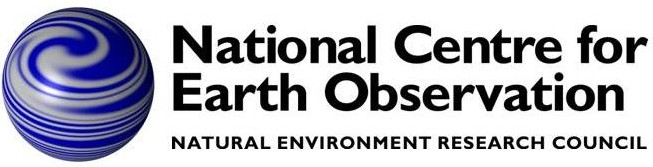# TIPS Data## Overview

Total Internal Partition Sum (TIPS) data are used to calculate temperature-dependent scale factors applied to line intensities. This is to allow for the fact that as the temperature increases, the ground state becomes depopulated as more molecules are raised to excited states.

If Q(T) denotes the TIPS value for temperature T, then, by definition, for absolute zero Q(0) = 1, and Q increases monotonically with temperature (the intensity scaling factor being 1/Q). However, it is a function which not only has to be calculated for each molecule, but also for each isotopologue of each molecule.

Since HITRAN line intensities are nominally defined for 296K, the scale factor applied for line intensity at temperature T is Q(296)/Q(T).

## History

TIPS data have been provided for 30 years or more by Gamache. In the original version (1980s?), Q(T) was generated for 0–3000 K using a 4th order polynomial (ie 4 coefficients for each molecule/isotope). The data were also provided in tabulated form at 1 K intervals.

With the release of HITRAN 2012 came a new representation of TIPS data tabulated at 25K intervals from 60–3010 K (so, 119 numbers per molecule/isotope) and a recommendation to use 4th order Lagrangian interpolation.

With HITRAN 2016 there is new formulation: the TIPS data are now tabulated at 1 K intervals from 1–20K, and 2K intervals above that, now with different upper temperatures for each molecule/isotope (ranging from 3500–9000K), so 1000s of numbers per molecule/isotope.

## RFM implementation

RFM v2
The original RFM implemented the 4th-order polynomial representation. However, there was also the option (now discontinued) to read in data tabulated at 1 K intervals and use this directly.
RFM v3, v4
With v3.0, the RFM implemented the 4th-order Lagrangian interpolation of the data tabulated at 25 K intervals. However, noting that only the ratio Q(T)/Q(296) is required, the original Gamache data were scaled by the value interpolated for 296K.
RFM v5
The full 2016 Gamache dataset is supplied via a subroutine which on its own is about a factor 3 larger than the entire RFM v5 source code. For this reason it was decided to subsample the data at 20K intervals and maintain the 4-point Lagrangian interpolation. The resulting data file tpsdat_dat.f90 is still the largest module within RFM v5 but about 20 times smaller than the original Gamache module (factor 10 from the subsampling, factor 2 from compressing Q into a single array for all molecules, isotopes).
TIPS data are provided for all molecules/isotopologues in the 2016 HITRAN dataset (and a few more: SO, C3H4, CH3 and CS2 — see
list of RFM absorbers). The only time the RFM is likely to encounter an unlisted molecule/isotopologue is when it's used with an alternative database such as GEISA (see GEIHIT for conversion). In these circumstances the RFM behaviour (set in modue qtfct_fnc.f90) is
• Unknown Isotopologue: default to use TIPS function for main isotopologue
• Unknown Molecule: default to assume Q(T) ~ (T/296)3/2 power law.